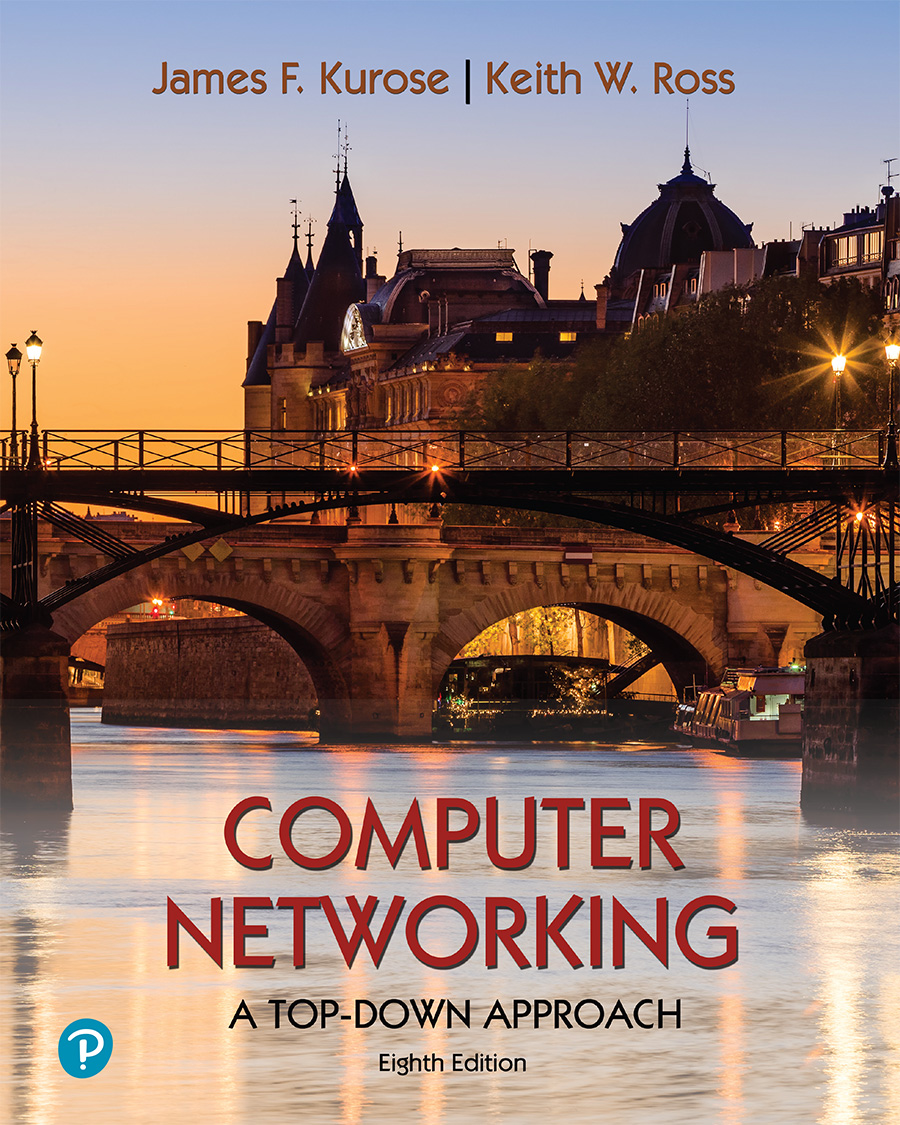# Interactive end-of-chapter exercises

Supplement to Computer Networking: A Top Down Approach 8th Edition

"Tell me and I forget. Show me and I remember. Involve me and I understand." Chinese proverb### Computing an Internet checksum

Consider the two 16-bit words (shown in binary) below. Recall that to compute the Internet checksum of a set of 16-bit words, we compute the one's complement sum  of the two words. That is, we add the two numbers together, making sure that any carry into the 17th bit of this initial sum is added back into the 1's place of the resulting sum); we then take the one's complement of the result. Compute the Internet checksum value for these two 16-bit words:

10111110   10100011      this binary number is 48803 decimal (base 10)

11000100   00000100      this binary number is 50180 decimal (base 10)

## Question 1 of 2

We gratefully acknowledge the programming and problem design work of John Broderick (UMass '21), which has really helped to substantially improve this site.